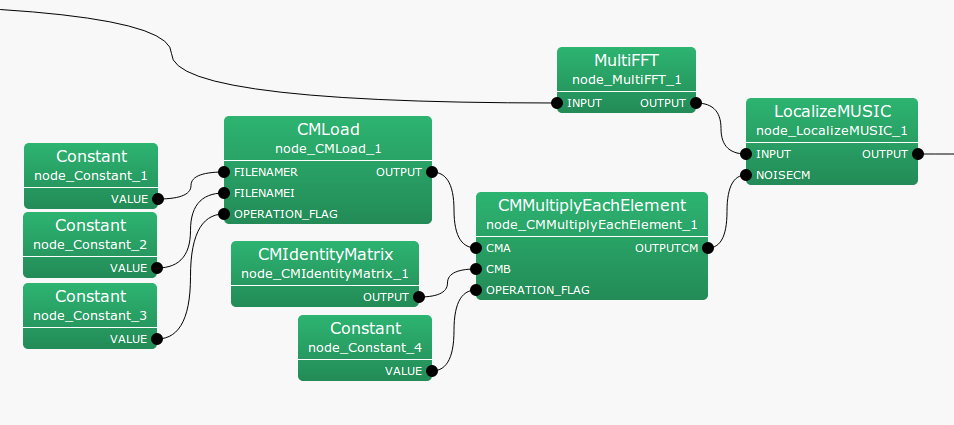6.2.7 CMMultiplyEachElement

6.2.7.1 Module Overview

Performs component-wise multiplication of two sound source correlation matrices.

None.

6.2.7.3 Usage

In what case is the node used?

The calculation node of the correlation matrix is the one created for the sound source from CMMakerFromFFT , CMMakerFromFFTwithFlag , and has the function of multiplying each component.

Typical Examples

Figure. 6.23 shows the usage example of CMMultiplyEachElement node.

The CMA input terminal is connected to a correlation matrix calculated from CMMakerFromFFT  or CMMakerFromFFTwithFlag , etc. (type is Matrix<complex<float> >  type, but to handle a correlation matrix, convert the three dimensional complex array to a two dimensional complex array and then output). Like CMA, the CMB input terminal also connects to the correlation matrix. At the time of multiplication, CMA $.*$ CMB is calculated, where $.*$ shows component-wise multiplication. OPERATION_FLAG is int  type, or bool  type input, and controls when the correlation matrix multiplication is calculated.Figure 6.23: Network Example using CMMultiplyEachElement

6.2.7.4 I/O and property setting of the node

Table 6.25: Parameter list of CMMultiplyEachElement
 Parameter Type Default Unit Description FIRST_FRAME_EXECUTION false Selection of the first frame execution ENABLE_DEBUG false ON/OFF of debugging information output

Input

CMA

: Matrix<complex<float> >  type. A correlation matrix for each frequency bin. The $M$-th order complex square array correlation matrix inputs $NFFT/2 + 1$ items. Matrix<complex<float> >  contains rows corresponding to frequencies ($NFFT/2 + 1$ rows), and columns containing the complex correlation matrix ($M * M$ columns across).

CMB

: Matrix<complex<float> >  type. Same as CMA.

OPERATION_FLAG

: int  type or bool  type. Only when this input terminal is 1 or when true, calculation of the correlation matrix is performed.

Output

OUTPUTCM

: Matrix<complex<float> >  type. The correlation matrix equivalent to CMA $.*$ CMB after multiplication is output.

Parameter

FIRST_FRAME_EXECUTION

: bool  type. Default value is false. When true, OPERATION_FLAG is always 0. Even when it is false, the operation is performed only on first frame.

ENABLE_DEBUG

: bool  type. Default value is false. When true, output the frame number calculated to the standard output,during correlation matrix division.

6.2.7.5 Module Description

Performs component-wise multiplication of each component of the two correlation matrices. Note that it is not a matrix multiplication of the correlation matrix (Refer to CMMultiplyMatrix  for matrix multiplication). The correlation matrix is a complex three-dimensional array of size $k \times M \times M$ and the multiplication $k \times M \times M$ times is performed as follows. Here, $k$ is the number of frequency bins ($k = NFFT/2 + 1$), and $M$ is the number of channels in the input signal.

OUTPUTCM = zero_matrix(k,M,M)

calculate{

IF OPERATION_FLAG
FOR i = 1 to k
FOR i = 1 to M
FOR i = 1 to M

OUTPUTCM[i][j][k] = CMA[i][j][k] * CMB[i][j][k]

ENDFOR
ENDFOR
ENDFOR
ENDIF

}

The matrix that is output from the OUTPUTCM terminal is initialized as a zero matrix, and maintains the final operational result from this point onward.